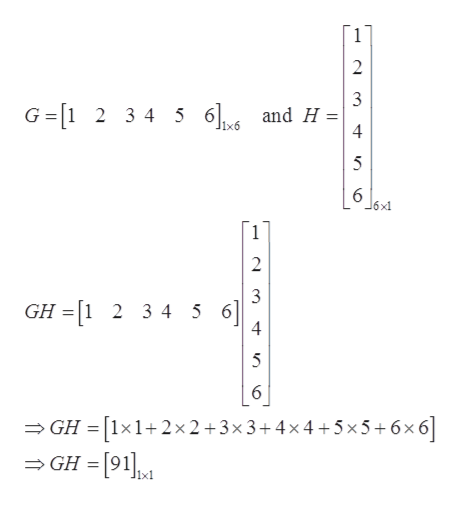# Given that G is a 1 × 6 matrix and H is a 6 × 1 matrix,a. Is GH defined? If so, what is the order of GH ?b.Is HG defined? If so, what is the order of HG?

Question
1 views

Given that G is a 1 × 6 matrix and H is a 6 × 1 matrix,

a. Is GH defined? If so, what is the order of GH ?

b.Is HG defined? If so, what is the order of HG?

check_circle

Step 1

Given that G is a 1 × 6 matrix and H is a 6 × 1 matrix,

a. Is GH defined? If so, what is the order of GH?

b. Is HG defined? If so, what is the order of HG?

Step 2

a.GH of order is 1 × 1

For example

...help_outlineImage Transcriptionclose2 G=[1 2 3 4 5 6]6 and H = 4 6x1 3 GH =[1 2 3 4 5 6 4 %3D 6 = GH = [1×1+2× 2+3×3+4x4 +5×5+6x6] = GH =  3. fullscreen

### Want to see the full answer?

See Solution

#### Want to see this answer and more?

Solutions are written by subject experts who are available 24/7. Questions are typically answered within 1 hour.*

See Solution
*Response times may vary by subject and question.
Tagged in

### Algebra